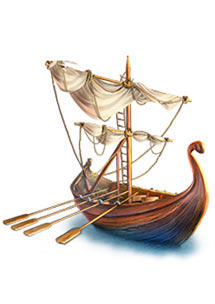Gold, Food and Supplies

Here is what each tile of gold, food and supplies gives you.

 GOLD Medallion = 4 units of gold Pearl = 5 units of gold Ring = 6 units of gold Bracelet = 7 units of gold Heart = 8 units of gold Helmet = 9 units of gold Pendant = 10 units of gold Turban = 11 units of gold Earring = 12 units of gold Chest = 14 units of goldFOOD Fish = 5 units of food Apple = 7 units of food Milk = 9 units of food  Lime = 11 units of food SUPPLIES Lumber = 5 units of supplies Brick = 9 units of supplies

Gold, Food and Supplies

Here is what each tile of gold, food and supplies gives you.

 GOLD Medallion = 4 units of gold Pearl = 5 units of gold Ring = 6 units of gold Bracelet = 7 units of gold Heart = 8 units of gold Helmet = 9 units of gold Pendant = 10 units of gold Turban = 11 units of gold Earring = 12 units of gold Chest = 14 units of goldFOOD Fish = 5 units of food Apple = 7 units of food Milk = 9 units of food  Lime = 11 units of food SUPPLIES Lumber = 5 units of supplies Brick = 9 units of supplies